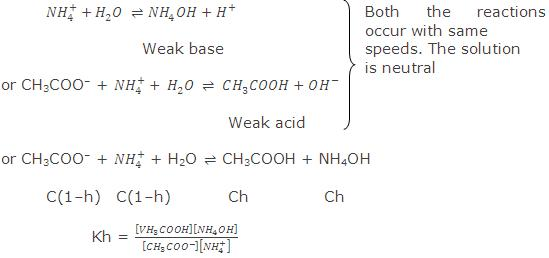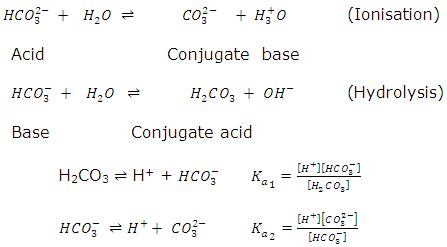# HYDROLYSIS OF SALTS

## Definitions

Salt is a compound formed by the neutralization reaction between an acid and a base. They generally ionize in water furnishing cations and anions. The cations or anions formed during ionization of salts either exist as hydrated ions in aqueous solutions or interact with water to regenerate the acids and bases. The process of interaction between cations or anions of salts and water is known as hydrolysis of salts. On the basis of hydrolysis, salts are divided into three categories:
• Acidic salts
•  Basic salts
• Neutral salts

Let us discuss hydrolysis of salts of the following types:

• Salts of strong acid and strong base: Salts formed by the neutralization of strong acid and strong base are neutral in nature as the bonds in the salt solution will not break apart. They generally get hydrated but do not hydrolyse. Therefore, such salts are generally known as neutral salts.

For example: NaCl

•  Salts of weak acid and strong base: Salts formed by the neutralization of weak acid and strong base are basic in nature. For example: CH3COONa

CH3COONa (aq)  CH3COO (aq) + Na+ (aq)

Acetate ion formed undergoes hydrolysis to form acetic acid and OH ions.CH3COO(aq) + H2O  Ch3COOH(aq) + OH(aq)
As we know acetic acid is a weak acid, it remains unionized in the solution. This results in an increase in concentration of OH  ions which makes the solution alkaline. pH of the solution is greater than7.

Salts of strong acid and weak base: Salts formed by the neutralization of strong acid and weak base are acidic in nature. For example: NH4Cl

NH4Cl(aq)  Cl(aq) + NH+4(aq)

Ammonium ion formed undergoes hydrolysis to form ammonium hydroxide and H+ ions.

NH+4(aq) + H2O  NH4OH(aq) + H+(aq)

As we know ammonium hydroxide is a weak base, it remains unionized in the solution. This results in an increase in concentration of H+ ions which makes the solution acidic. pH of such solutions is less than 7.
Salts of weak acid and weak base: Salts formed by the neutralization of weak acid and weak base are acidic, basic or neutral depending on the nature of acids and bases involved. For example: CH3COONH4.General mechanism for the hydrolysis of ions formed from these salts:

CH3COO + NH+4 + H2O  CH3COOH + NH4OH

Degree of hydrolysis in such cases is independent of the concentration of solution and pH of such solutions is given by:

pH= 7 + 12 (pKa – pKb)

Hence, we can say that the pH of a solution can be less than 7 or greater than 7 depending on the values of pKa and pKb.• An ion is a charged atom that is made from losing or gaining electrons.
• hydroxide ion (OH-) is produced when the conjugate base accepts a proton from water and causes the solution to become basic
• conjugate acid is a substance formed when the base gains a hydrogen ion.
• conjugate base is a substance formed when the acid loses a hydrogen ion.
• hydronium ion (H3O+) is produced when the conjugate acid donates a proton to water, causing the solution to become acidic.

Now we have enough review of terms to understand salt hydrolysis, which is when salt completely dissociates in water and its anion or cation reacts with the water to produce hydroxide ions or hydronium ions that affect the pH of the solution.

## Salts of Strong Acids and Weak Bases

Salt formed from a strong acid and a weak base will make an acidic solution when added to water. The reason this occurs is because when the salt dissociates, the conjugate acid of the weak base will donate a proton, or one hydrogen ion, to water and form hydronium. NH4NO3 is a salt that comes from a strong acid, HNO3, and a weak base, NH3. When added to water, the salt dissociates into NH4+ and NO3- ions. The NH4+ ion, which is a conjugate acid of the weak base, reacts with water by donating one hydrogen to water and forming hydronium.

Let’s look at these equations to see how the salt dissociates and how the conjugate acid of the weak base, or the ammonium ion, reacts with water:

NH4NO3(s)<–>NH4+(aq) + NO3-(aq)

NH4+(aq) + H20(l) <–> NH3(aq) + H3O+(aq)

With more hydronium ions (H3O) in the water, the solution becomes more acidic than basic.

• ## Salt of a Strong Acid and a Weak Base

The solution of such a salt is acidic in nature. The cation of the salt which has come from weak base is reactive. It reacts with water to form a weak base and H+ ions.

B+  +   H2O    BOH  +  H+

Weak base

Consider, for example, NH4Cl. It ionises in water completely into NH4 and CF ions. ions react with water to form a weak base (NH4OH) and H+ ions.

NH+4  +  H2O      NH4OH  +  H+

C(1-x)                   Cx         Cx

Thus, hydrogen ion concentration increases and the solution becomes acidic.

Applying law of mass action,

…… (i)

where C is the concentration of salt and x the degree of hydrolysis.

Other equilibria which exist in solution are

NH4OH  NH+4 + OH,

…. (ii)

H2  H+ + OH,

Kb = [H+][H]            ….. (iii)

From eqs. (II) and (iii)

Kw/Kb =[H+ ][NH4 OH]/[NH4+ ] = Kh      …. (iv)

[H+] = [H+ ][NH4+]/[NH4OH] = Kw/Kb ×[NH4+ ]/[NH4 OH]

log [H+] = log Kw – log Kb + log[salt]/[base]

-pH = -pKw + pKb + log[salt]/[base]

pKw – pH = pKb + log[salt]/[base]

### Relation Between Hydrolysis Constant and Degree of Hydrolysis

The extent to which hydrolysis proceeds is expressed as the degree of hydrolysisand is defined as the fraction of one mole of the salt that is hydrolysed when the equilibrium has been attained. It is generally expressed as h or x.

h = (Amount of salt hydrolysed)/(Total salt taken)

Considering again eq. (i),

Kh = x2C/(1-x)   or     Kh = h2C/(1-h)

When h is very small (1-h) → 1,

H2 = Kh × 1/c

or   h = √(Kh/C)  = √(Kw/Kb  C)

[H+] = h × C = √(C  Kh)/Kb

log [H+] =  1/2 log Kw + 1  1/2log C – 1/2log Kb

### Salt of a Weak Acid and a Strong BaseThe solution of such a salt is basic in nature. The anion of the salt is reactive. It reacts with water to form a weak acid and OH ions.

A + H2O;      HA + OH

Weak acid

Consider, for example, the salt CH3COONa. It ionises in water completely to give CH3COO and Na+ ions. CH3COO ions react with water to form a weak acid, CH3COOH and OH ions.

CH3COO + H2O     CH3COOH + OH

C(1-x)                         Cx           Cx

Thus, OH ion concentration increases, the solution be­comes alkaline.

Applying law of mass action,

Kh = [CH3COOH][OH]/[CH3CO] = (Cx×Cx)/C(1-x) = (Cx2)/(1-x) )  …… (i)

Other equations present in the solution are:

CH3COOH  CH3COO + H+,

Ka = [CH3COO][H+]/[CH3COOH]      …… (ii)

H2  H+ + OH,

Kw = [H+][OH]    ……. (iii)

From eqs. (ii) and (iii),

log [OH] = log Kw – log Ka + log[salt]/[acid]

-pOH = -pKw + pKa + log[salt]/[acid]

pKw – pOH = pKa + log[salt]/[acid]

Considering eq. (i) again,

Kh = cx2/(1-x)      or    Kh = Ch2/(1-h)

When h is very small, (1-h) → 1

or     h2 = Kh/C

or     h =  √Kh/C

[OH] = h × C = √(CKh) = √(C  Kw/Ka)

[H+] = Kw/[OH]

= Kw/√(C*Kw/Ka) = √(Ka Kw)/Kc

-log [H+] = -1/2log Kw – 1/2log Ka + 1/2log C

pH =  1/2pKw +  1/2pKa +  1/2log C

= 7 + 1/2pKa +  1/2log C.

### Salt of a Weak Acid and a Weak BaseMaximum hydrolysis occurs in the case of such a salt as both the cation and anion are reactive and react with water to produce H+ and OFT ions. The solution is generally neutral but it can be either slightly acidic or slightly alkaline if both the reactions take place with slightly different rates. Consider, for example, the salt CH3COONH4. It gives CH3COO and  ions in solution. Both react with water.Other equilibria which exist in solution are:

CH3COOH   CH3COO + H+,

Ka =  [CH3COO][H+]/[CH3COOH]    ….. (i)

NH4OH  NH+4 + OH,

Kb = [NH+4] [OH]/[NH4OH]           ….. (ii)

H2 H+ + OH

Kw = [H+][OH]                                    ….. (iii)

From Eqs. (i), (ii) and (iii),

Kh =  Kw/Ka.Kb = [CH3COOH][NH4OH]/[CH3COO][NH+4]                     …. (iv)

Let C be the concentration and h be the degree of hydrolysis

Kh = h2/(1-h)2

When h is small, (1-h) → 1.

Kh = h2

h = √Kh = √Kw/Ka*Kb

[H+] Ka × h

= Ka × √Kw/Ka*Kb

= √K Ka/Kb

-log [H+] = -1/2log Ka – 1/2log Kw + 1/2log Kb

pH = 1/2pKa +  1/2pKw – 1/2pKb

= 7 +  1/2pKa – 1/2pKb

When pKa = pKb, pH = 7, i.e., solution will be neutral in nature.

When pKa > pKb. The solution will be alkaline as the acid will be slightly weaker than base and pH value will be more than 7.

In case pKa < pKb, the solution will be acidic as the acid is relatively stronger than base and pH will be less than 7.

Salt of a Strong Acid and a Strong Base

Such a salt, say NaCl, does not undergo hydrolysis as both the ions are not reactive. The solution is thus, neutral in nature.

• ## Hydrolysis of Amphiprotic Anion

Let us consider hydrolysis of amphiprotic anion only, i.e., when counter cation is not hydrolysed, example of some salts of this category are NaHCO3, NaHS, Na2HPO4, NaH2PO4.Here, H2PO4 and HPO2-4  are amphiprotic anions. pH after their hydrolysis can be calculated as,

pH of  H2PO4  in aqueous medium = (pka1 + pka2)/2

pH of H2PO2-4  in aqueous medium = (pka2 + pka3)/2

Here, H2PO2- is conjugate base of H2PO4 and H3PO4 is conjugate acid of H2PO4.

Similarly, PO3-4 is conjugate base of HPO-24  and HPO4  is conjugate acid of PO3-4 .

(iv)  Let us consider amphiprotic bicarbonate anion.pH HCO3of  ion after hydrolysis in aqueous medium  = (pka1 + pka2)/2

(v)  Let us consider the hydrolysis of amphiprotic anion along with cation, e.g., NH4HCO3, NH4HS.

In above examples both cations and anions are derived from weak base and weak acids respectively hence, both will undergo hydrolysis in aqueous medium.

When these salts are dissolved in water, [H3O+] concentra­tion can be determined as,

[H3O+] = √ka1[kw/kb + ka2]

pH = -log = √ka1[kw/kb + ka2]

## Hydrolysis at a Glance

 Salt Nature Degree Hydrolysis Constant pH NaCl (Strong acid + Strong Base)   2. Ch3COONa (Weak acid + Strong base)   3. NH4Cl (Strong acid + Weak base)   4. CH3COONH4   (Weak     acid + Weak base) Neutral       Base     Acidic     * No Hydrolysis       h = √kw/Cka     h = √kw/Ckb     h = √kw/(ka + kb) –       Kh = kw/ka     Kh = kw/Ckb     Kh = kw/(ka + kb) –       pH=1/2[pkw + pka + logC]   pH=1/2[pkw- pkb – logC]     pH=1/2[pkw + pka – pkb]

In the case of salt of weak acid and weak base, nature of medium after hydrolysis is decided in the following manner:

(i)    If Ka = Kb, the medium will be neutral.

(ii)  If Ka > Kb, the medium will be acidic.

(iii)  If Ka < Kb, the medium will be basic.

The degree of hydrolysis of salts of weak acids and weak bases is unaffected by dilution because there is no concentration term in the expression of degree ofhydrolysis.

Note : Degree of hydrolysis always increases with increase in temperature because at elevated temperature increase in Kw is greater as compared to Ka and Kb.

## STEM Elearning

We at FAWE have built this platform to aid learners, trainers and mentors get practical help with content, an interactive platform and tools to power their teaching and learning of STEM subjects, more